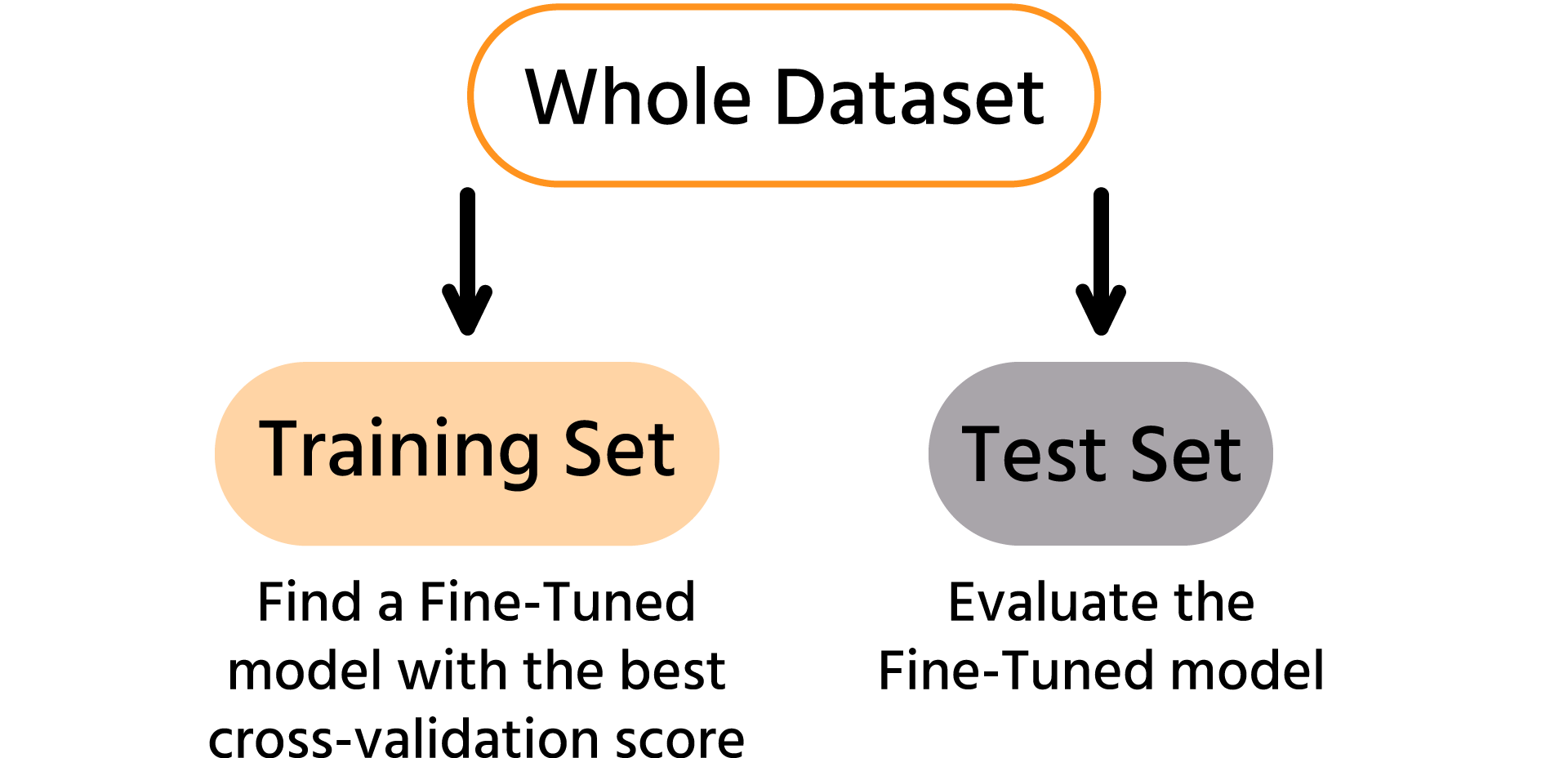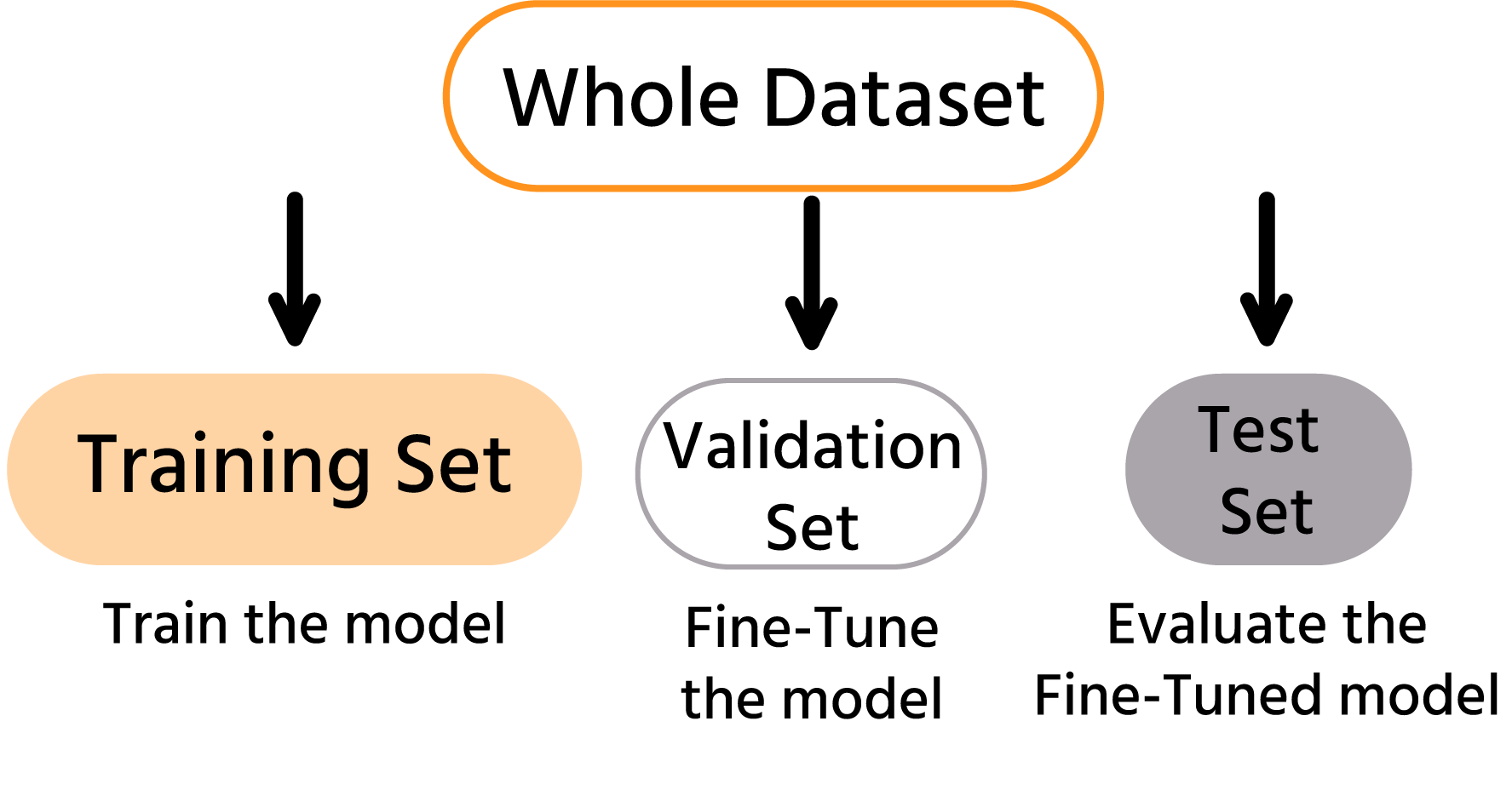Course Content

ML Introduction with scikit-learn

##Modeling Summary

Congratulations on getting so far! You already know how to build a model, use it in a pipeline, and fine-tune the hyperparameters! You also learned two ways to evaluate the model: the train-test split and the cross-validation score.
Let's talk about combining model evaluation and hyperparameter tuning performed by `GridSearchCV` (or `RandomizedSearchCV`).

Note

Since our dataset is tiny, we will use the `GridSearchCV`, but everything said below also applies to a `RandomizedSearchCV`.

In general, we want to get the best cross-validation score on the dataset because a cross-validation score is more stable (less sensitive to how we split) than the train-test split.
So we want to find the hyperparameters leading to the best cross-validation score (precisely what `GridSearchCV` does).

We end up with the fine-tuned model that performs best on this specific set of instances (the training set). We also get the cross-validation score since `GridSearchCV` provides `.best_score_` calculated while finding the best hyperparameters.
The problem is that the best hyperparameters on a specific dataset are not guaranteed to be the best in general for your problem. If new data is added to the dataset – the best hyperparameters might change.
So the `.best_score_` may be better than the score on completely unseen data because hyperparameters that are best on one dataset may be good but not the best for new data.
Usually, the dataset is first split into train-test sets. Then fine-tune the model on a whole training set to the cross-validation score. Once we find the best-tuned model, we evaluate its performance on completely unseen data, a test set.Let's sum it all up. We need:

1. Preprocess the data.
2. Do a train-test split.
3. Find the model with the best cross-validation score on the training set.
This includes trying several algorithms and finding the best hyperparameters for them.
To simplify, we only used one algorithm in this course.
4. Evaluate the best model on the test set.

That's what you will do in the next chapter! It includes all the steps you have learned throughout the course to assemble it into a final pipeline.

Before moving on to the final challenge, it needs to be mentioned that using cross-validation to fine-tune the model is not the only way.
As the dataset grows larger, it takes much more time to calculate the cross-validation score, and the regular train-test split becomes more stable since a test set is larger.

That's why a large dataset usually is split into 3 sets: a training set, a validation set, and a test set.
This way, we train the model on a training set and evaluate the performance on a validation set. We do it for different models/hyperparameters to find the model with the best score on the validation set.
So while finding the fine-tuning model, we use a validation set's score instead of a cross-validation score. And for the same reason, we need to evaluate it then on completely unseen data, test set.Our Penguins dataset is not large. It is actually tiny (342 instances), so we will use the cross-validation score approach in the next chapter.

Everything was clear?

Section 4. Chapter 9